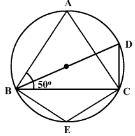# MCQ Questions for Class 9 Maths Chapter 10 Circles with Answers

Students can access the NCERT MCQ Questions for Class 9 Maths Chapter 10 Circles with Answers Pdf free download aids in your exam preparation and you can get a good hold of the chapter. Use MCQ Questions for Class 9 Maths with Answers during preparation and score maximum marks in the exam. Students can download the Circles Class 9 MCQs Questions with Answers from here and test their problem-solving skills. Clear all the fundamentals and prepare thoroughly for the exam taking help from Class 9 Maths Chapter 10 Circles Objective Questions.

## Circles Class 9 MCQs Questions with Answers

Students are advised to solve the Circles Multiple Choice Questions of Class 9 Maths to know different concepts. Practicing the MCQ Questions on Circles Class 9 with answers will boost your confidence thereby helping you score well in the exam.

Explore numerous MCQ Questions of Circles Class 9 with answers provided with detailed solutions by looking below.

Question 1.
If there are two separate circles drawn apart from each other, then the maximum number of common points they have:
(a) 0
(b) 1
(c) 2
(d) 3

Question 2.
D is diameter of a circle and AB is a chord. If AD = 50 cm, AB = 48 cm, then the distance of AB from the centre of the circle is
(a) 6 cm
(b) 8 cm
(c) 5 cm
(d) 7 cm

Question 3.
In a circle with center O and a chord BC, points D and E lie on the same side of BC. Then, if∠BDC=80°, then ∠BEC =
(a) 80°
(b) 20°
(c) 160°
(d) 40°

Question 4.
The center of the circle lies in______ of the circle.
(a) Interior
(b) Exterior
(c) Circumference
(d) None of the above

Question 5.
If chords AB and CD of congruent circles subtend equal angles at their centres, then:
(a) AB = CD
(b) AB > CD
(d) None of the above

Question 6.
Segment of a circle is the region between an arc and ………..of the circle.
(a) perpendicular
(c) chord
(d) secant

Question 7.
In the figure, triangle ABC is an isosceles triangle with AB = AC and measure of angle ABC = 50°. Then the measure of angle BDC and angle BEC will be(a) 60°, 100°
(b) 80°, 100°
(c) 50°, 100°
(d) 40°, 120°

Question 8.
The region between chord and either of the arc is called
(a) a sector
(b) a semicircle
(c) a segment
(d) a quarter circle

Question 9.
The region between an arc and the two radii joining the centre of the end points of the arc is called a:
(a) Segment
(b) Semi circle
(c) Minor arc
(d) Sector

Question 10.
If a line intersects two concentric circles with centre O at A, B, C and D, then:
(a) AB = CD
(b) AB > CD
(c) AB < CD
(d) None of the above

Question 11.
A chord of a circle which is twice as long as its radius is a ____ of the circle
(a) Diameter
(b) perpendicular
(c) arc
(d) secant

Question 12.
A regular octagon is inscribed in a circle. The angle that each side of the octagon subtends at the centre is
(a) 45°
(b) 75°
(c) 90°
(d) 60°

Question 13.
Equal _____ of the congruent circles subtend equal angles at the centers.
(a) Segments
(c) Arcs
(d) Chords

Question 14.
The angle subtended by the diameter of a semi-circle is:
(a) 90
(b) 45
(c) 180
(d) 60

Question 15.
The degree measure of a semicircle is
(a) 0°
(b) 90°
(c) 360°
(d) 180°

Question 16.
In the given figure if OA = 5 cm, AB = 8 cm and OD is perpendicular to AB, then CD is equal to(a) 4 cm
(b) 3 cm
(c) 5 cm
(d) 2 cm

Question 17.
AB is a chord of a circle with radius ‘r’. If P is any point on the circle such that ∠APB is a right angle , then AB is equal to
(a) 3r
(b) r
(c) 2r
(d) r2

Question 18.
In a circle with center O and a chord BC, the point D lies on the same side BC as O. If ∠ BOC = 50°,then ∠ BDC =
(a) 25°
(b) 100°
(c) 75°
(d) 150°